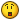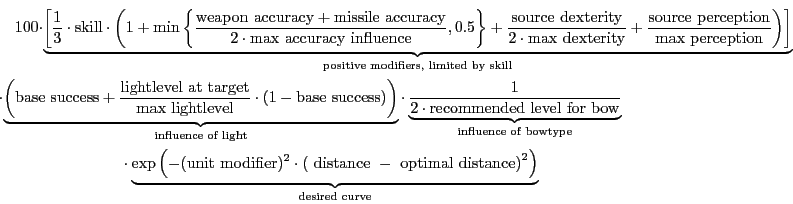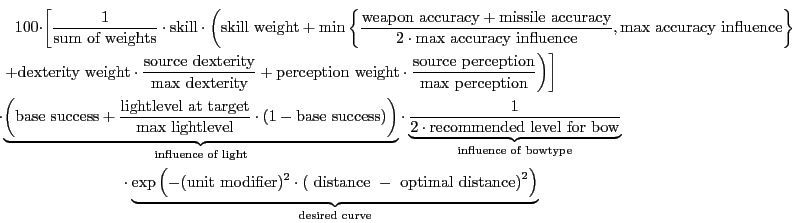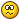Eternal Lands Official Forums

## Recommended Posts

I would like to get some feedback on the chance to hit with an arrow. If you are good with math, take a look at this post.

We have the following parameters:

Parameters that add to the chance:

weapon and missile accuracy

source player dexterity

source player perception

source perception

source ranged skill

Parameters that diminish from the chance:

target player reaction

distance to target (squared)

light level of the target (0 means very dark, 256 is fully lit)

The formula should return a number from 0 to 100, which will be the chance in percent to hit.

So, if you feel like you came up with a good formula, please post it here.

##### Share on other sites

(accuracy*(dexterity*10 + perception*10) + skill * 2) / 7 - ((t_reaction*5 + distance ^ (1/3)) + (light_level / 256 * 100))

Assuming real math.

Edited by freeone3000

##### Share on other sites

hmm.

((weapon and missle accuracy*5)/(distance to target^2))+(source player dexterity - target player reaction)+(source player perception*(light level of the target/2))+source ranged skill/5

Edited by Ryokotsusei

##### Share on other sites

distance to target (squared)

I'm curious...would different weapon have different ranges?

Shortbow (300 pixels)

Longbow (500 pixels)

Compoundbow (800 pixels)

so if you are using a Compoundbow..and target is 400 pixels away, then 50/50 chance to hit

but if using Shortbow......................................................................... 0/100%

and finally Longbow.........(range of weapon*2-distance*2)/10 = 20% chance to hit

##### Share on other sites

distance to target (squared)

I'm curious...would different weapon have different ranges?

Shortbow (300 pixels)

Longbow (500 pixels)

Compoundbow (800 pixels)

so if you are using a Compoundbow..and target is 400 pixels away, then 50/50 chance to hit

but if using Shortbow......................................................................... 0/100%

and finally Longbow.........(range of weapon*2-distance*2)/10 = 20% chance to hit

As a suggestion: It might be nice to have the Shortbow have good short range accuracy, with poor long-range accuracy... BUT also make the type of bow best at long range have some sort of penalty for short range shots. This would prevent all but one type of bow from becoming useless.

##### Share on other sites

I would like to get some feedback on the chance to hit with an arrow. If you are good with math, take a look at this post.

We have the following parameters:

Parameters that add to the chance:

weapon and missile accuracy

source player dexterity

source player perception

source perception

source ranged skill

Parameters that diminish from the chance:

target player reaction

distance to target (squared)

light level of the target (0 means very dark, 256 is fully lit)

The formula should return a number from 0 to 100, which will be the chance in percent to hit.

So, if you feel like you came up with a good formula, please post it here.

Could you provide some more information about what variables have more importance. Maybe a 'weighting' or ranking of sorts? For example: would the attackers dexterity be more important then the defenders reaction? That might help with generating the formula.

##### Share on other sites

(accuracy*(dexterity*10 + perception*10) + skill * 2) / 7 - ((t_reaction*5 + distance ^ (1/3)) + (light_level / 256 * 100))

Assuming real math.

The skill should be more important than the attributes, and the formula should return a number from 0 to 100...

Could you provide some more information about what variables have more importance. Maybe a 'weighting' or ranking of sorts? For example: would the attackers dexterity be more important then the defenders reaction? That might help with generating the formula.

The most important bias should be, in this order:

skill, distance, weapon/misisle accuracy, light level, then the attributes.

In fact, the light level should be combined with the distance.

##### Share on other sites

Could you provide some more information about what variables have more importance. Maybe a 'weighting' or ranking of sorts? For example: would the attackers dexterity be more important then the defenders reaction? That might help with generating the formula.

The most important bias should be, in this order:

skill, distance, weapon/misisle accuracy, light level, then the attributes.

In fact, the light level should be combined with the distance.

OK, this helps a lot. One other thing that might be needed would be a range for some the variables, namely lighting and distance (perhaps a range of the weapon/missle accuracy.) Those seem to be needed to make sure the resulting number falls into the 0-100 range. The other ranges are known, of course (attributes and skill level but might be nice for those that aren't sure...)

##### Share on other sites

OK, this helps a lot. One other thing that might be needed would be a range for some the variables, namely lighting and distance (perhaps a range of the weapon/missle accuracy.) Those seem to be needed to make sure the resulting number falls into the 0-100 range. The other ranges are known, of course (attributes and skill level but might be nice for those that aren't sure...)

You could just normalize the ones with unknown range in your formula, i.e. value -> value/max_value

##### Share on other sites

distance to target (squared)

I'm curious...would different weapon have different ranges?

Shortbow (300 pixels)

Longbow (500 pixels)

Compoundbow (800 pixels)

so if you are using a Compoundbow..and target is 400 pixels away, then 50/50 chance to hit

but if using Shortbow......................................................................... 0/100%

and finally Longbow.........(range of weapon*2-distance*2)/10 = 20% chance to hit

When I read this, I jumped up and started searching the net.

Chances go from 0 - 100% so, the answer is in statistics (the curve of Gauss etc.)

But the off-set curve (to the left and or to the right) can give better accuracy for close distance, or high distance.

Take a look at these plots, it says it all:

off-set Graphs to the left and or to the right (in the middle is the normal curve of Gauss - bit strange)

The formula for getting these off-set plots is:

off set formula

where a positive outcome stands for close distance accuracy (bump of graph on the left side)

and a negative outcome stands for far distance accuracy (bump of graph on the right side)

The mathematicians amongst us know what the "sigma X" and the "n" represent, but for the non-mathematicians a short list:

Ex (sigma x) = add all the possible outcomes

n = the total numbers you added together.

example average of 1+2+3+4+5+6 =

Ex -> 21

--- -> ---- = 3,5

n -> 6

That's in short what the parts of the equation mean ... now you can hussle them and multiply it to power 3.

(My mathematical-english isn't that good, but I mean x^3 with power 3)

I dont know how you can put the variables we are looking for into the equations, but I am sure there is a way to add the skill, distance+light, weapon/missile accuracy and attributes in these formulas so we get the normal Gauss curve and the 2 off set curves, where the distance on the curve = the distance to target, while we keep the height of the curve from 0 - 100.

I hope my fellow mathematicians know what I am talking about##### Share on other sites

I played around a bit and needed to introduce a "recommended level" for bows, which makes sense I'd say. Also didn't know what "source perception" is, compared to "source player perception".

My formula contains another additional parameter to make up for "bow type" as mentioned above (determines an "optimum range").

` 100*skill*(1+MIN( (weapon_accuracy+missile_accuray)/20; 0.5) + source_dexterity/96 + source_perception/48) * EXP(-(distance-optimumdistance)^2) * (0.5+light_target/512) * 1/(3*2*recommended_level_bow)`

I introduced the factor 3 at the end there because the first factor is limited by 3. I left out an exeption handling system if the target distance is the same as the optimum weapon distance, that factor should be set to 1 in that case (this isn't necessary if you don't want to specialize bows like that). The factor 20 at the weapon and missile accuracy is semi-arbitraryset it to (weapon_accuracy + missile_accuracy) that's supposed to be the maximum influence of this factor.

One more thing, you'll have to use the minimum of this and 100 or else you'll get values higher than 100 (because I don't want to assume skill level to be bounded by 178, I think a recommended level for bows makes more sense here).

And of course it would be nice to penalize less for distances closer to you....

edit: you might want to introduce a factor to modify the distance penalty, i.e. replace (distance-optimumdistance)^2 with a^2(distance-optimumdistance)^2

edit2: played around a bit, so here's a more readable versionand here's a generalization:edit3: Taylor series had an error in it, just used exp() now, as I was told there are fast implementations for it anyways.

edit4: last one, I promise: there was a real brain fart in the formula, fixed now.

Edited by Ermabwed

##### Share on other sites

OK, this helps a lot. One other thing that might be needed would be a range for some the variables, namely lighting and distance (perhaps a range of the weapon/missle accuracy.) Those seem to be needed to make sure the resulting number falls into the 0-100 range. The other ranges are known, of course (attributes and skill level but might be nice for those that aren't sure...)

You could just normalize the ones with unknown range in your formula, i.e. value -> value/max_value

I agree, it would be easy enough to do this way but it would also be nice to be able to test the results with some numbers that make sense. Just plugging in random numbers to get an answer seems a bit weak in the testing of such a formula.

Edited by DogBreath

##### Share on other sites

OK, this helps a lot. One other thing that might be needed would be a range for some the variables, namely lighting and distance (perhaps a range of the weapon/missle accuracy.) Those seem to be needed to make sure the resulting number falls into the 0-100 range. The other ranges are known, of course (attributes and skill level but might be nice for those that aren't sure...)

You could just normalize the ones with unknown range in your formula, i.e. value -> value/max_value

I agree, it would be easy enough to do this way but it would also be nice to be able to test the results with some numbers that make sense. Just plugging in random numbers to get an answer seems a bit weak in the testing of such a formula.

Well, you could pick a reasonable parameter and limit the influence and leave it to ent to modify it, compare what I did with the missile and weapon accuracy in my post above for an example. I limit the influence of this parameter to half the influence of the skill level at the most, so you won't be able to shoot well with the überbow when your skill level is too low to use it.

The important part of this formula as I see it, is modifying a desired curve (giving the chance to hit the target) with the parameters involved in a reasonable way. I chose a bell-curve and for example the chance to hit a target that is in complete darkness is half of that to hit when in complete light, if you wanted it to be a third you'd have to modify that part, etc.

##### Share on other sites

I'll use this notation:

q = weapon and missile accuracy

d = source player dexterity

p = source player perception

s = source ranged skill

Parameters that diminish from the chance:

w = target player reaction

r = distance to target (not squared)

L = light level of the target (0 means very dark, 1 is fully lit)

alpha, beta, gamma, ... - unknown coefficients to determine by experiment.

Formula returns to hit probability (0 <= p <= 1).

Assume that player has the sphere form of radius R. Then if it doesn't move to-hit probability

should be

P_0 = erf ( R/r * (alpha*s + beta*d + gamma*p) )

Target reaction is more difficult to emulate, and I have no idea how to include lighting in the formula,

but I think they are connected: reaction determines how far can target move while arrow is flying.

While lighting determines how well we can see where it is going to move.

For example, they can be combined this way: w * (delta - epsilon*L).

And here is absolutely wrong formula how to combine P_0 and target reaction,

but it least it keeps the main idea of what is going on:

P = P_0 * (1 - erf (w * (delta - epsilon*L) )

Finally about optimal distance: it shouldn't be included in to-hit probability formula.

Obviously if target is right in front of you you'll not miss.

But bows that can shot at long distance are usually hard, and time between shots

would be longer, that for short ranged bows. This might prevent them from

becoming useless.

##### Share on other sites

Here's my formula, hope it'll help:

```D = (square_dist_max - square_dist) / (square_dist_max - square_dist_min)
P = 1 - (256 - light) * (48 - perception) / (256 * 48)

hit = (skill + accuracy + 12 + (dexterity - reaction) / 4) * (P + D) / 1.5```

Note 1: as the distance is squared, the decrease of precision will be more important when the distance is high.

Note 2: the final factor (1.5) can be modified according to the maximum skill a player can reach and the maximum accuracy he can have.

I've done some tests and the formula seems to work well. The only problem is that if a player max all his attributes and has a very good archery level (around 100), he will have more than 100% of chance to hit his target on short distances. But it's normal as I've designed the formula in order he can almost reach 100% at max distance if he has the max to all attributes and an archery level around 100.

##### Share on other sites

How about one that decreases the chance of hit exponentially?

Let

S=skill lvl

D=distance from target

A=accuracy (note: for the formula to work, accuracy must be the accuracy lvl from the minimum of accuracy one could get ingame. I believe -8 is the minimum by wearing full bronze and no moon med)

L=light lvl

T=dexterity

R=target reaction

e=exp function

c=general constant to help low lvl training (i liked it around 10 or so)

General formula:

sqrt((S^2.05)+(T^2)+L-(R^2)-256+c(100-S)) all divided by

e^(D/A)

Y = sqrt((S^2.05)+(T^2)-(R^2)+L-256+5(150-S)) all divided by

e^(D/5A)

Percent Hit = minimum of either Y or 100.

The last part of the numerator c(100-S) 5(150-S) is a term I added as I worked with the formula to allow extremely low lvl trainers to hit. As one lvls up, this term will approach zero. However, we must pick a c, which i have chosen 5, such that the term S^2.05 increases much greater than 100c thus it doesnt pay off to remain at low lvl. This formula works well if the max lvl is capped at 100 which could be done since it would be a new skill.

Some data points when your dext is equal to target's reaction and accuracy is 8 higher than the minimum accuracy ingame with a full lighting (256):

At distance 8, the hit rate would be:

skill ------ hit

100 ------ 41.27 98.8

90 ----- 37.23 90.3

50 ------ 21.88 59.4

40 ------- 18.48 53.1

20 ------ 13.08 43.7

10 ------- 11.7 41.3

5 -------- 11.49 40.8

1 -------- 11.58 40.7

If you are interested in this formula, I have made an excel2003 sheet on this and could email it to you. Otherwise, good luck with the formula and I hope you will find one that fits well.

EDIT: After checking to see how far 6 steps are ingame, I felt that 6 steps were shorter than i thought. So the formula has been changed slightly.

Edited by Lesbegue

##### Share on other sites

Alia and me talked about this a bit last evening and that made me sit down again do some additional thinking to include a high chance to hit close targets and the fact that the maximal range of a shot is limited by a constant i.e. initial velocity squared, divided by g; this is basically a weapon parameter which I'll call max_range.

To bring the chance at this maximal range down to zero we can use exp(-1/a(r-max_range)^2), where "a" is a parameter that basically lets us determine how fast we want it to go down (r denotes the distance), i.e. multiply the function of the (base) curve by

```1						  if r <= max_range
exp(-1/(a(r-max_range)^2)) if r > max_range.```

I don't know if there you have better cut off functions in some libraries but this does the job anyways and allows really skilled players (depending on the factor "a" some possible miracle shots tooNow as for the rest of the curve, we'll have to compare some graphs and see what you want there, I'll be looking at modifying another bell curve (as will be Alia I believe).

Generally I believe for a very skilled player at the optimal conditions the curve should tend towards

```100 if r <= max_range
0   if r > max_range```

where the cut off would be taken care of by the above mentioned function.

For an unskilled player at worst conditions the curve should drop very fast, basically the chance to hit a target one tile (if you can shoot at targets next to you, else make that 2 tiles) away under these conditions should be very small (10% perhaps) and drop to less than say 1% at greater distances (2 (or 3) tiles and more).

##### Share on other sites

For those who posted formulas, would it be possible to generate some graphs with different parameters and stuff in order to have a more visual thingy?

It's kind of hard to try to visualise the formulas in your brain, especially since some of them are quite complex.

##### Share on other sites

I made an generic spreadsheet with some scrollbars to modify the various attributes and a sample graph (no serious contribution to the task at hand, just showing how you can get it plotted for a single variable (I used distance), modifying the parameters with the scrollbars will cause the graph to be plotted again.

Have fun: bowshit.ods (right click and "save as...")

##### Share on other sites

I think you have your axes revesed?

Anyway, I tried playing with the parameters, but the chances only modify for the very close distances (under 10 tiles). Further away, the chances are always under 1-2%

##### Share on other sites

I think you have your axes revesed?

Anyway, I tried playing with the parameters, but the chances only modify for the very close distances (under 10 tiles). Further away, the chances are always under 1-2%

Well, that formula in there is just a placeholder, the spreadsheet is meant as a tool for others to test their formulas. I'll hopefully be able post a better formula than the one I posted above some time today.

Oh and the file name is to be read as "bows hit"##### Share on other sites

LOL

I was thinking: hmm, this guy names his files pretty much the way I do tooBut anyway, are you sure the axes are not reversed?

##### Share on other sites

Oh, axes lol, I was wondering what the heck you were talking about, were we going to be able to use axes as ranged weapons as well?They are correct I think (A column as x-axis, B column as y-axis), I hate these diagramsIt looks like they are wrong...I'll play around with the whole thing a bit more and post some sample formulas...

oh and here's an Excel version that hopefully works: bowshit.xls

just a quick edit: RL's calling, I'll work on this later todayEdited by Ermabwed

##### Share on other sites

Here are some graphs extracted from my formula. I didn't played with modifiers like accuracy, dexterity and reaction because they are directly added to skill so they don't have a big impact on the face of the curve. The factors that change the face of the curves the most are the skill, the distance, the light and the perception and are represented on the following graphs.

I just recall the formula before:

```D = (square_dist_max - square_dist) / (square_dist_max - square_dist_min)
P = 1 - (256 - light) * (48 - perception) / (256 * 48)
hit = (skill + accuracy + 12 + (dexterity - reaction) / 4) * (P + D) / 1.5```Note: I've assumed that the shortest distance is 2 meters and the longest is 50 meters.

You can notice that on all the graphs, if a player with a low perception try to hit someone who is far and in a dark place, he has almost no chance to touch him whatever are his other attributes. In the formula, the perception is used to correct the loss of precision when the light is low but don't add any advantages when the light is full.

And again, the global factor of 1.5 at the end of the formula can be changed to a better one according to the max skill and accuracy a player can reach...

I can make more graphs if needed.

Edited by Schmurk

##### Share on other sites

Ok, I've looked at the spreadsheet again and it seems to work as intended (tested by plotting some basic functions).

After playing around a bit I modified my first formula to: (use in B31 of the spreadsheet, then copy&paste to B32:B130), the factor "20" is intended as a bow parameter (see my post above), I think it's different from general weapon accuracy (compare: sniper rifle has high accuracy but only a skilled rifleman can use it properly).

`=IF(A31>=max_r; 0; MIN(100; 100*skill*(1+MIN( (m_acc+w_acc)/20; 0.5)+((spd-tpr)/96)+(spp/48) )*( 0.5+(ll/512))*EXP( -((A31)^2)/shape^2)/(6*20)))`

This IF-function of course is just a very crude version of a cut off function as outlined aboveAnd one more thing: you want to fix the y-axis of the plot (click on it (it gets (green) markers, right mouse click select properties, scale, disable automatic and set minimum to 0, maximum to 100).

I'm still counting on Alia to post a version that moves some of the parameters into the exponential function.